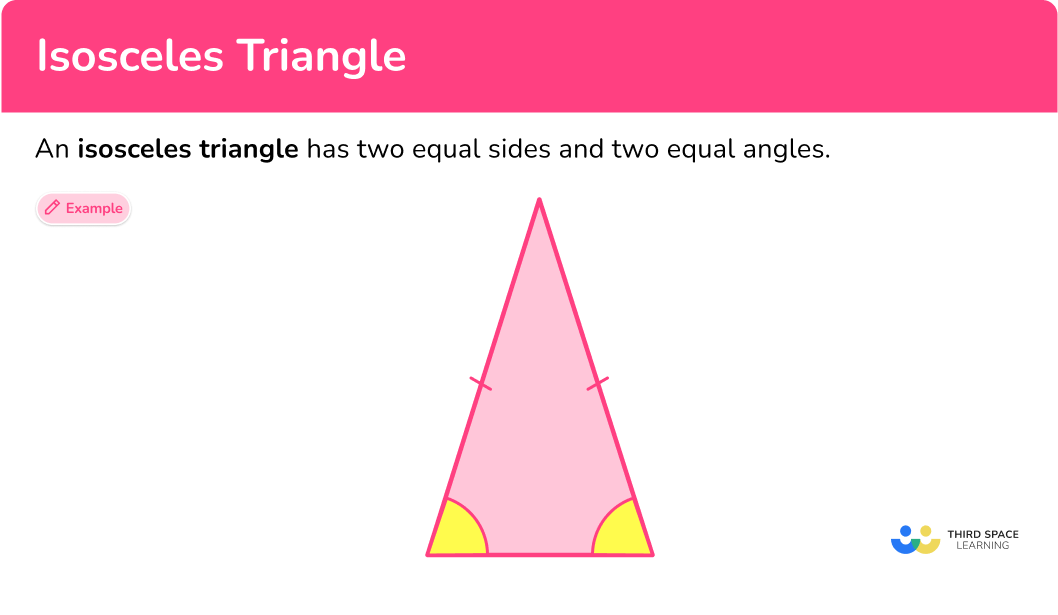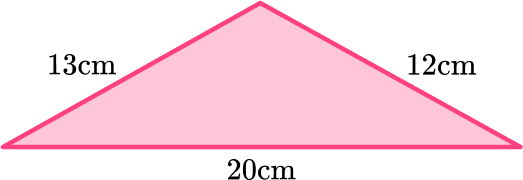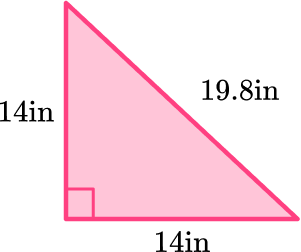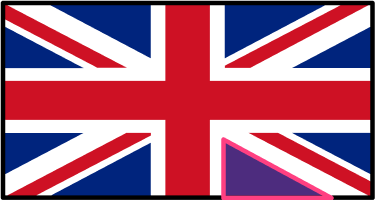Math resources Geometry Triangles

Isosceles triangle

# Isosceles triangle

Here you will learn about isosceles triangles, including what an isosceles triangle is and the properties of isosceles triangles.

Students first learn about triangles in kindergarten and 1 st grade in geometry with their work in reason with shapes and their attributes. They expand their knowledge of triangles as they progress through elementary school and into secondary school.

## What is an isosceles triangle?

An isosceles triangle is a unique type of triangle because it has two equal (congruent) sides and two equal (congruent) angles.

Properties of an isosceles triangle:

• Two sides of equal length.
• Two equal angles.

The dashes on the sides and the angles of the triangle mean they are equal in measure or congruent.

The equal (congruent) angles of an isosceles triangle are called the base angles.

The base angles are opposite the equal (congruent) side lengths.

The unequal side (third side) of an isosceles triangle is often called the base of the triangle.

The unequal angle (third angle) of an isosceles triangle is called the vertex angle.

There are three different types of isosceles triangles.

Obtuse isosceles triangleAcute isosceles triangleRight isosceles triangle
● Has 1 obtuse angle

● Has 3 acute angles

● Has 1 right angle

### Symmetry

An isosceles triangle has \bf{1} line of symmetry. The line goes from the vertex to the exact middle of the base. The line of symmetry is perpendicular to the base of the isosceles triangle.

### What is an isosceles triangle?## Common Core State Standards

• Grade 4 – Geometry (4.G.A.3)
Recognize a line of symmetry for a two-dimensional figure as a line across the figure such that the figure can be folded along the line into matching parts. Identify line-symmetric figures and draw lines of symmetry.

• Grade 5 – Geometry (5.G.B.3)
Understand that attributes belonging to a category of two-dimensional figures also belong to all subcategories of that category.

## How to identify isosceles triangles

In order to identify isosceles triangles:

1. Recall the definition.
2. Explain why the triangle fits or does not fit the definition.

## Isosceles triangle examples

### Example 1: classify isosceles triangles

Is the triangle below an isosceles triangle?

1. Recall the definition.

An isosceles triangle is a triangle with two equal (congruent) sides and two equal (congruent) angles.

2Explain why the triangle fits or does not fit the definition.

This triangle is an isosceles triangle because it has two side lengths that are 12 \, cm each. So, the triangle has two equal (congruent) sides.

### Example 2: classify isosceles triangles

Is the isosceles triangle below a right isosceles triangle, obtuse isosceles triangle, or acute isosceles triangle?

An obtuse isosceles triangle is an isosceles triangle that has 1 obtuse angle.

An acute isosceles triangle is an isosceles triangle that has 3 acute angles.

A right isosceles triangle is an isosceles triangle that has 1 right angle (90^{\circ}).

The isosceles triangle shown has one 100^{\circ}, which is an obtuse angle. So, the triangle is an obtuse isosceles triangle.

### Example 3: classify isosceles triangles

Choose the triangle that is a right isosceles triangle.

A right isosceles triangle is an isosceles triangle with one right angle, (90^{\circ}).

Triangle A is not the right choice. Triangle A has 1 right angle, but it is not an isosceles triangle because there are no side lengths that are the same.

Triangle C is not the right choice. Triangle C is an isosceles triangle because it has two sides with lengths that are equal (congruent), but it is not a right triangle.

Triangle B is the correct choice because it has two side lengths that are equal (congruent) and it has a right angle, so it is a right isosceles triangle.

### Example 4: symmetry isosceles triangle

How many lines of symmetry does the triangle have?

Isosceles triangles have one line of symmetry going through the vertex angle to the exact middle of the base.

This isosceles triangle has 1 line of symmetry that goes through the vertex angle to the middle of the base of the triangle.

### Example 5: classify isosceles triangle

What type of isosceles triangle is shown in this image?

An obtuse isosceles triangle is an isosceles triangle that has 1 obtuse angle.

An acute isosceles triangle is an isosceles triangle that has 3 acute angles.

A right isosceles triangle is an isosceles triangle that has 1 right angle (90^{\circ}).

The banners in the photo appear to be acute isosceles triangles because the vertex angle and the base angles appear to be acute angles, greater than 0^{\circ} and less than 90^{\circ}.

### Example 6: classifying isosceles triangles

What type of isosceles triangle does this photo represent?

An obtuse isosceles triangle is an isosceles triangle that has 1 obtuse angle.

An acute isosceles triangle is an isosceles triangle that has 3 acute angles.

A right isosceles triangle is an isosceles triangle that has 1 right angle (90^{\circ}).

The hanger appears to be an obtuse isosceles triangle because the vertex angle appears to be obtuse, greater than 90^{\circ} and less than 180^{\circ}.

### Teaching tips isosceles triangles

• Incorporate drawing activities where students can use rulers and protractors to create isosceles triangles.

• Incorporate origami activities that are creative and help students understand the properties of triangles, specifically isosceles triangles.

• Although worksheets have their place for practicing skills, using digital platforms for game playing is fun for students and helps them to practice skills. Check out free platforms such as desmos, quizlet, blooket, and quizziz.

### Easy mistakes to make

• Thinking that isosceles triangles can be classified as equilateral triangles
A question may ask students to explain if an isosceles triangle can be equilateral. The easy mistake to make is stating that isosceles triangles can be classified as equilateral triangles. Understanding that properties of isosceles triangles and equilateral triangles can help with questions like this.

Isosceles triangles only have two equal (congruent) sides and angles and cannot be classified as equilateral. However, equilateral triangles have three equal (congruent) sides and angles and can be classified as isosceles.

• Angle facts
A common mistake when classifying triangles is mixing up the definitions of acute angle and obtuse angle. So when students classify the triangles, they wind up classifying them incorrectly. Knowing angle facts will help with this.
• Acute angles are greater than 0^{\circ} and less than 90^{\circ}.
• Right angles equal 90^{\circ}.
• Obtuse angles are greater than 90^{\circ} and less than 180^{\circ}.

• Base angles
The common mistake is thinking that the base of the angles are always on the bottom of the isosceles triangle. Seeing the triangles in different positions will help with this understanding.

For example, here is a picture where the base angles of an isosceles triangle are on the top.

• Sides of equal length
The common mistake is identifying the wrong sides as the equal (congruent sides). Make sure that you get the equal sides and angles in the correct position. It is the 2 sides which are opposite the 2 equal base angles which are equal in length.

### Practice isosceles triangle questions

1. Which of these diagrams shows an isosceles triangle?The dash marks on the sides of the triangle indicate which lengths are equal. You need the triangle which has the same mark on two sides.

2. How many lines of symmetry does an isosceles triangle have?

0123An isosceles triangle only has one line of symmetry that cuts the triangle into two equal parts that are mirror images of each other.

The line of symmetry goes from the vertex angle to the middle point of the base.3. Select the triangle that represents an obtuse isosceles triangle.An obtuse isosceles triangle is an isosceles triangle that has 1 obtuse angle.4. If a triangle has side measurements of 14 inches, 14 inches, and 19.8 inches and one angle that is 90^{\circ}, what type of triangle is it?

Isosceles triangleRight isosceles triangleAcute isosceles triangleRight scalene triangleHere is a drawing of the triangle with the given information.The triangle has two side lengths that are equal in measure, and it also has a 90^{\circ}. So, the triangle is a right isosceles triangle.

5. Which photo represents an isosceles triangle?An isosceles triangle has two equal sides and two equal angles.

This photo of the roof appears to be an isosceles triangle because of the two equal sides and the two equal angles.6. Joey drew a picture of an isosceles triangle. Which set of angle measurements can be the angles of the triangle she drew?

90^{\circ}, 55^{\circ}, 35^{\circ}100^{\circ}, 45^{\circ}, 35^{\circ}90^{\circ}, 60^{\circ}, 30^{\circ}70^{\circ}, 55^{\circ}, 55^{\circ}An isosceles triangle has two equal (congruent) sides and two equal (congruent) angles.

So the angle measurements of the triangle Joey drew could be 70^{\circ}, 55^{\circ}, 55^{\circ}.

## Isosceles triangle FAQs

How do you find the area of isosceles triangles?

You can use the formula for finding the area of a triangle, which is a=\cfrac{1}{2} \cdot b \cdot h. The height of an isosceles triangle is a line segment that starts at the vertex angle and is perpendicular to the opposite side. The height is also known as the altitude of the triangle.

How do you find the perimeter of the isosceles triangle?

You can find the perimeter of an isosceles triangle the same way you would find the perimeter of any polygon, add up all the side lengths.

Does an isosceles triangle have a hypotenuse?

Only right isosceles triangles will have a hypotenuse. The hypotenuse is the longest side of a right triangle, so only right triangles have hypotenuses.

What is trigonometry?

Trigonometry is the study of triangles where you will learn ratio relationships of right triangles such as sine, cosine, and tangent ratios. You will learn about trigonometry in high school.

Can you use the Pythagorean theorem on isosceles triangles?

You can only use the Pythagorean theorem on right isosceles triangles because the Pythagorean theorem only applies to right triangles.

## Still stuck?

At Third Space Learning, we specialize in helping teachers and school leaders to provide personalized math support for more of their students through high-quality, online one-on-one math tutoring delivered by subject experts.

Each week, our tutors support thousands of students who are at risk of not meeting their grade-level expectations, and help accelerate their progress and boost their confidence.